Q.1. Distinguish among dia, para and ferromagnetic substance.

Ans ⇒

 S.N Diamagnetic Substances Paramagnetic Substances Ferromagnetic Substances 1. Found in all three states. Also found in three states. Crystalline solids. 2. When suspended in a magnetic field try to set them ⊥’ to magnetic field. When suspended in a magnetic field, set parallel to magnetic field or try to set. When suspended in magnetic field they come to parallel the magnetic field. 3. Value of μ is less than 1. μ is much greater than 1. μ is much greater than 1. 4. Value of K is negative and small. The value of K is small but positive. K is positive and large. 5. No effect of temp. on their susceptibitlity. Magnetic susceptibility is inversely proportional to the absolute temperature i.e Ka On heating above Curie temp. they are converted in paramagnetic. 6. There is no Curie point. No fixed Curie point. Their Curie temp is fixed. 7. Do not exhibit hysteresis. Do not exhibit hysteresis. Exhibit hysteresis.

Q.2. (a) Describe in brief terrrestrial magnetism.
(b) Discuss modern therory of magnetism. (c) Compare a bar magnet & solenoid.

Ans ⇒ (a) Earth’s or Terrestrial Magnetism : Earth behave like a huge magnet. The axis of magnetic needle suspended freely indicates the magnetic force field. Magnetic poles do not coincide with geographical poles. The physical quantities which specify the magnitude & direction of magnetic field at a place are called magdnetic elements. Magnetic elements are following there :
(i) Declination : At a place, angle between geographical meridian & magnetic meridian is called declination. It is espessed Gsθ° east or 0° west ata place.

(ii) Dip or inclination : At a particular place angle made by magnetic force field of earth with horizontal is called dip. or dip angle or inclination. Its value changes from place to place.

(iii) Horizontal intensity : At a place in magnetic meridian the horizontal component of magnetic force field is called horizontal intensity.

(b) Modern therory of magnetism : The atomic model of magnet is concerned with atomic structure. In each atom there is a positive charged nucleus around which electrons revolve in different orbits. The orbital motion of electron is equivalent to an elementry current.
So it was thought that due to orbital motion of electron magnetism is produced & there is magnetic moment. Besides orbital motion there is spin motion of electron so magnetic moment is also exist. So net magnetic moment is resultant of the two magnetic moment.
In most of atom of materials there are such pair of electrons whose orbital & spin motion are in opposite direction so net magnetic moment is zero. Such materials are if placed in external magnetic field then they are not magnetised in the direction of field. These are called non magnetic substance. Besides it due to different electronic arrangement there are existence of diamagnetic, paramagnetic & ferromgnetic substances. Due to special setting of atoms in materials small domains are set on increasing temp. these domains are scattered.

(C) Compare Bar Magnet & Solenoid : At the centre of a circular conductor a magnetic force field is set up normally in plane of circle. If such circular conductors are set one after other on axis of circular conductor such that axis is ⊥r to their planes & pass through their centres and in all conductors current are clockwise & anticlock wise then near the axis &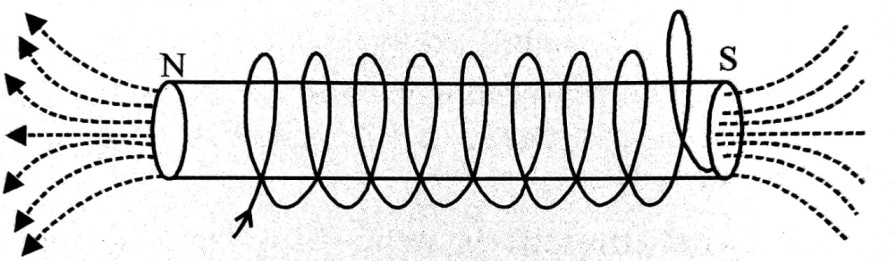parallel to axis a long magnetic field is produced on glass or card board insulating wire is warapped in spiral shape then it is called solenoid.
On left end north pole is produced while on right end south pole is produced the strength of magnet is fixed but magnetic field strength due to solenoid can be increased or decreased by changing the strength of electric current on reversing the direction of current the polarity is reversed.

Q.3. Give the theory of oscillation magnetometer. How the magnetic moments of two bar magnets are compared dithout meassuring moment of inertia.

Ans ⇒ Theory : When a magnet is suspended freely in a uniform field, using an unspun thread, it comes to rest in a direction NS parallel to direction of field. If this magnet is slightly deflected from its equilibrium position and left, the magnet begins to vibrate simple harmonically about the direction of the field as tis mean position.

Construction : Oscillation magnetometer is a rectangular box made of wood. The four sides and the top contain glass plates. From the cenre of the upper lid a hollow cylindrical pipe (V) is fitted. At the top of it there is a hook H. From the hook a silk theread is attached whose other and contains a stirrup made of brass S. In this stirrup a maghnet way be balanced in horizontal plane. At the end of the piep there is a torsion head T. In order to cout the oscilliation of the magnet there are two windows W1 and W2 in the top lid. At the bottom there is a glas plate on which refrence line has been drawn. Looking at the image of the magnet in the plane mirror and the line drawn on it, the oscillation can be counted correctly.

Method : If the means of determining moment of inertia is not available then the magnets are made to oscillate jointly and then moments are compared, without meauring moment of inertia. First both the magnets are placed together on the stirrup with similar poles togther and then with dissimilar poles together.

The corresponding time periods are T1 and T2 respectively are determined. if the moments of the magnets be M1 and M2 and M1 > M2 then is the first condition the effecting magnetic moments will be (M1 + M2) and second case (M1 – M2) since the magentic moments are vector quantities.
Both the magnets oscillate together and therefore in both cases the moment of inertia will be I = (I1 + I2) since they are scalar quantities. Hence,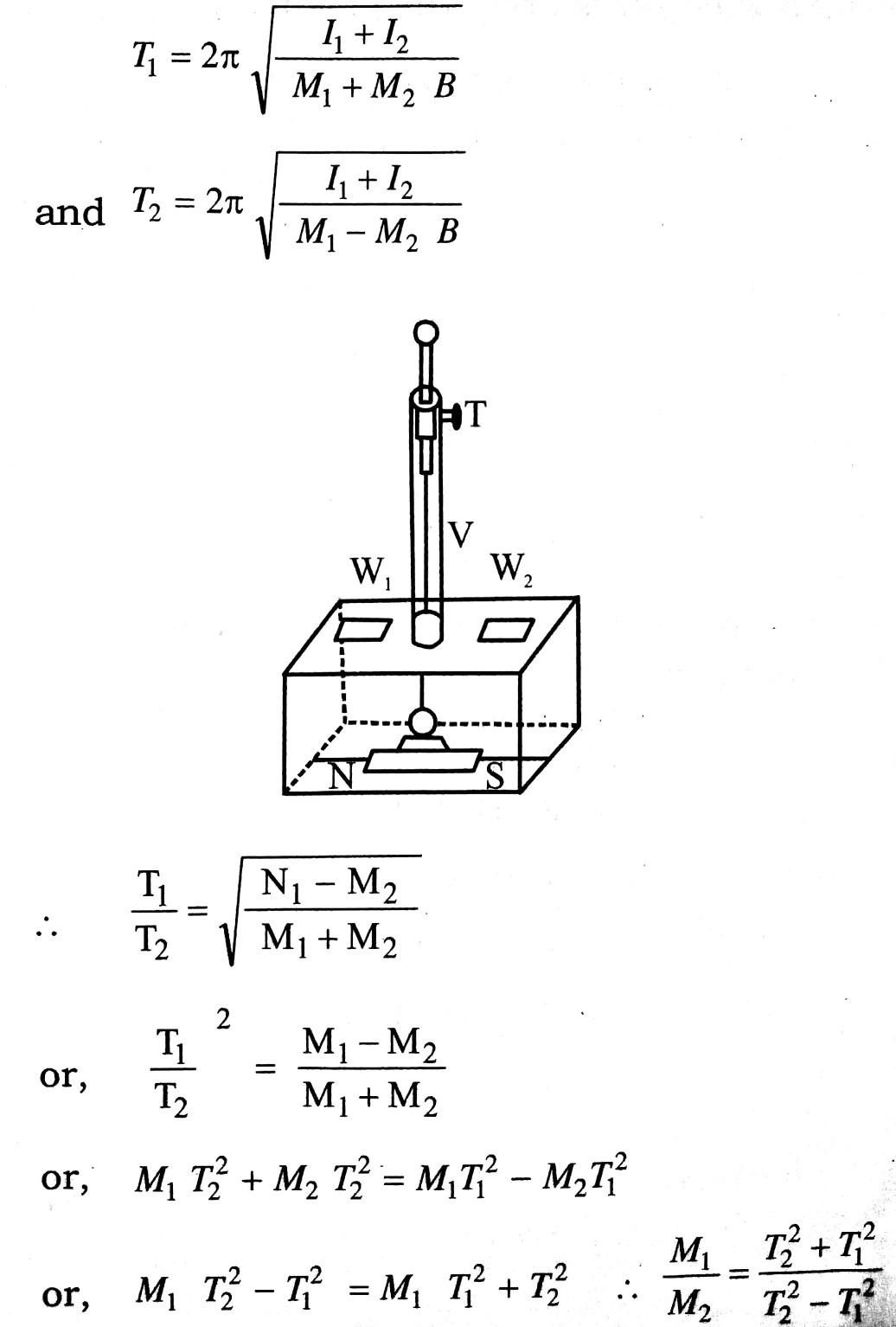Procedure : Before using this apparatus it is made horizontal by means of the screws S1 S2 and S3. Then with the help of a compass needle the scratch in the plane mirror is brought along the merdian. A brass piece is placed on the stirrup ot remove torsion in the thread. After sometime the torsion is removed and the brass piece stands in a certain position. Then with the help of torsion head the brass piece is brought in line with the meridian by making it parallel to the scratch. Afterwards the brass piece is removed by hand and a magnet with its north pole towards the north and S-pole towards the south is kept on the stirrup.

Q.4. (a) Derive an expression for the torque on a freely suspended magnet on a uniform magnetic field.
(b) Calculate the work done in the deflection of a magnet in a uniform magnetic field.

Ans ⇒ (a) Torque on a magnet :
NS is a bar magnet of pole strength pand length 2l. It is suspended freely in a uniform magnetic field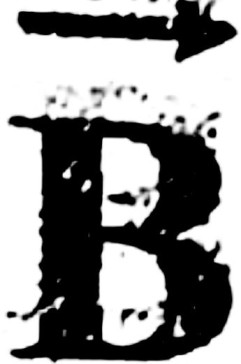. When it is deflected from the direction ofby an

angle θ, the forces (p, p) act on its two poles which are equal, opposite and parallel. Hence a couple is formed which tr is bring the magnet in the direction of. This couple is called centrolling couple. The moment of couple or torque is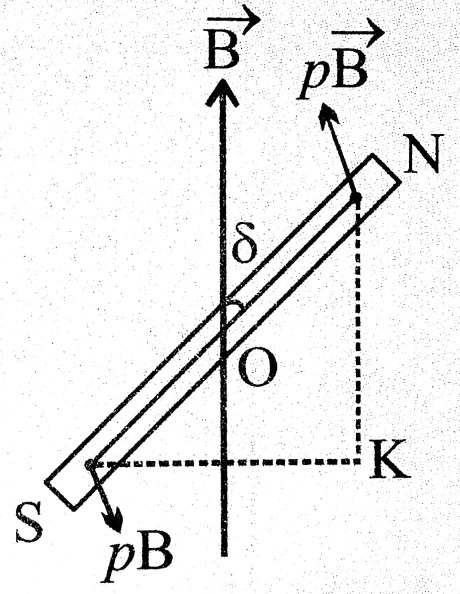τ = one force x perpendicular distance between the two forces
= pB x Sk
= pB x 2l sinθ
= (p. 2l)B sinθ

 ⇒                τ = mBsinθ                            …(i)

where m = p.2l = magnetic moment of magnet.
Since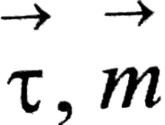andall are vectors.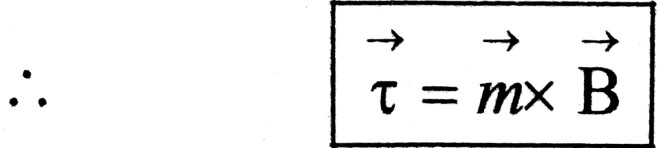… (ii)

(b) Work done in the deflection of a magnet in a uniform magnetic field :
We consider a magnet SN of magnetic moment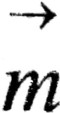suspended freely in a uniform magnetic field. When it is deflected form the direction of magnetic fieldby an angle φ, the moment of entrolling couple acting on the magnet is given by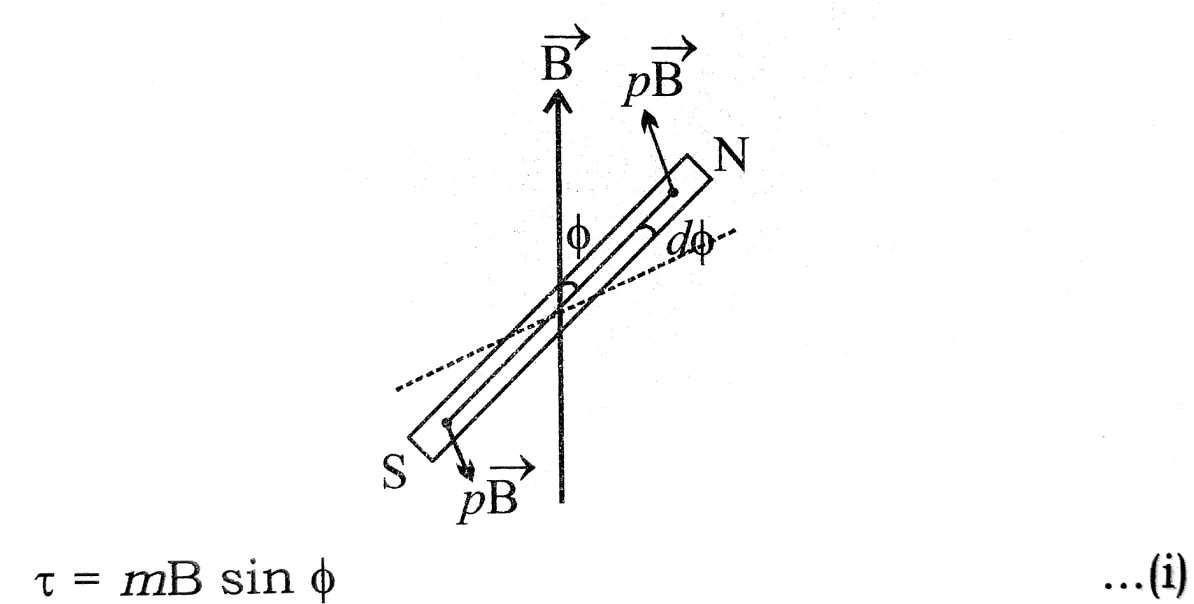If the deflection is increased by a small angle dφ, work done against the couple is
dW = torque x angular displacement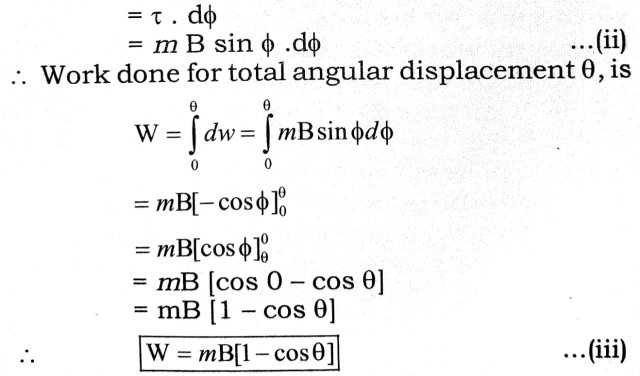This is the required work done.

Q.5. Prove that a bar magnet is equivalent to a current carrying solenoid.

Ans ⇒ Solenoid as a Bar magnet :
We know that the existence of a magnetic field is due to a moving charge or due to an electric current. In this context according to magnetic effect of current, each current loop behaves as a magnetic dipole having magnetic dipole moment.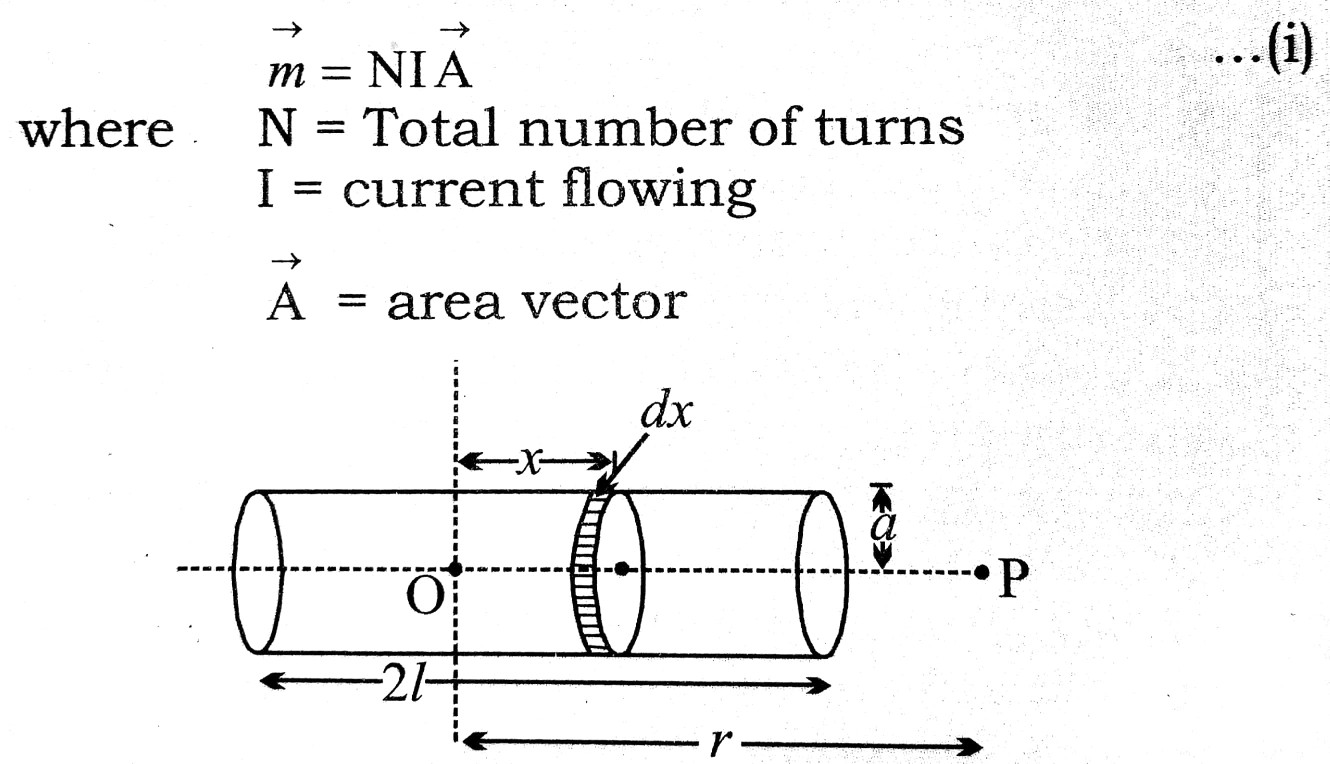Let for current carrying current solenoid (fig.)
Radius of circular section = a
Length of solenoid = 2l
Total number of turns = N

Number of turns per unit length n = N/2l
Current flowing in solenoid = I
A small element of solenoid of thickness dx at a distance x from the centre of the solenoid is a circular coil. Magnetic field at any point P on the axis of the circular coil is given by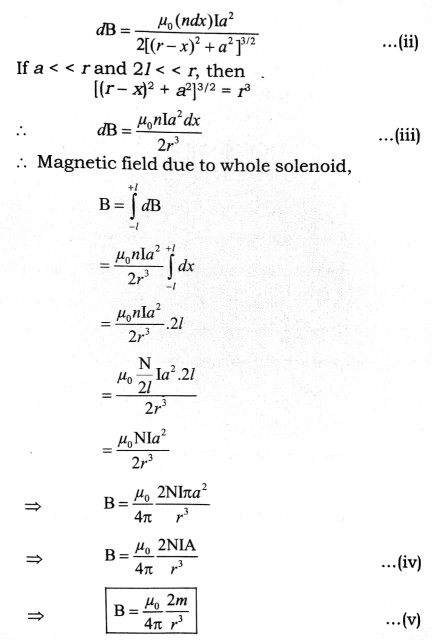The magnetic field on the axis of a small magnet is just equal to the value in eq. (v). It proves the equivalence of solenoid and a bar magnet.

## Class 12th physics Long Type question in English

 S.N Physics Long Type Question English Medium 1. ELECTRIC CHARGES AND FIELDS 2. LECTROSTATIC POTENTIAL AND CAPACITANCE 3. 4. 5. MAGNETISM AND MATTER 6. ELECTROMAGNETIC INDUCTION 7. ALTERNATING CURRENT 8. ELECTROMAGNETIC WAVES 9. RAY OPTICS AND OPTICAL INSTRUMENTS 10. WAVE OPTICS 11. DUAL NATURE OF MATTER AND RADIATION 12. ATOMS 13. NUCLEI 14. SEMI CONDUCTOR ELECTRONICS 15. COMMUNICATION SYSTEMS
You might also like